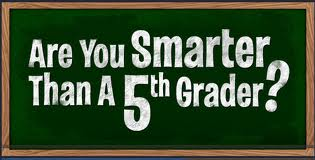# Are you smarter than a fifth grader?A 2009 digit number $N$ is multiplied by 54. The last two digits of this product are 68. When $N$ is multiplied with 46, find the last two digits.

Note- This question is taken from a class 5 Math talent exam paper, thus the title.

×